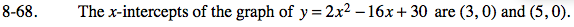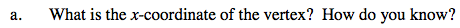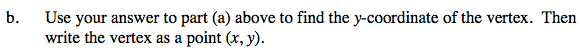### Home > CAAC > Chapter 8 > Lesson 8.2.3 > Problem8-68

8-68.The x coordinate of the vertex is halfway between 3 and 5.

x = 4Substitute the vertex value of x back into the original equation to find y.

y = 2(42) − 16(4) + 30

Solve for y.

y = −2

Coordinates = (4, −2)